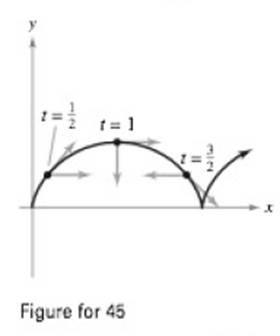Chapter 12.4, Problem 49E

Chapter
Section
Textbook Problem
24 views

# Cycloidal Motion The figure shows the path of a particle modeled by the vector-valued function r ( t ) = ( π t − sin π t , 1 − cos π t ) .The figure also shows the vectors v ( t ) / ‖ v ( t )     a n d   a ( t ) / ‖ a ( t ) ‖ at the indicated values of t(a) Find a 1 and a 2 at t = 1 2 , t = 1 ,   and   t = 3 2 (b) Determine whether the speed of the particle is increasing or decreasing at each of the indicated values of t Give reasons for your answers.(a)

To determine

To Calculate: Find aT and aN at t=112, t=1 and t=3/2

Explanation

Given: The provided vector-valued function is,

r(t)= πtsinπt, 1cosπt

The path represents by the function is shown below,

Formula Used:

The tangential and normal component of the acceleration, and magnitude of the vector are shown below,

aT=v.avif r(t)=a i+b j+c k||r(t)||=a2+b2+c2an=v×av

Calculation:

Now, find the the velocity vector of the particle s shown below,:,

v(t)=r|(t)=ππcosπt, πsinπThe speed of the vector,  v = π2(1cosπt)2+π2sinπt)=π12cosπt+cos2πt+sin2πt=π12cosπt+1 [as sin2 πt+cos2πt=1]=π12cosπt=π2(1cosπt)=π2×2sin2 πt2= 2πsinπt2The acceleration vector of the particle is a(t)=r||(t)=π2sinπt, π2cosπtNow, the tangetial component of acceleration,aT=v.av= ππcosπt,πsinπt.π2sinπt,π2cosπt2πsinπt2=π(1cosπt)sinπt+π3sinπ+cosπ2πsinπt2=π2sinπt2sinπt2

Now |v×a | = |i j kππcosπt πsinπt 0π2sinπt <

(b)

To determine

To Calculate: Whether the speed of the particle is increasing or decreasing at each of the indicated value of t.

### Still sussing out bartleby?

Check out a sample textbook solution.

See a sample solution

#### The Solution to Your Study Problems

Bartleby provides explanations to thousands of textbook problems written by our experts, many with advanced degrees!

Get Started

#### Differentiate the function. H(z)=a2z2a2+z2

Single Variable Calculus: Early Transcendentals, Volume I

#### [(18)1/3]2

Applied Calculus for the Managerial, Life, and Social Sciences: A Brief Approach

#### For and , a × b =

Study Guide for Stewart's Multivariable Calculus, 8th

#### In Exercises 2124, find the distance between the points. (3,2) and (9,7)

Finite Mathematics for the Managerial, Life, and Social Sciences